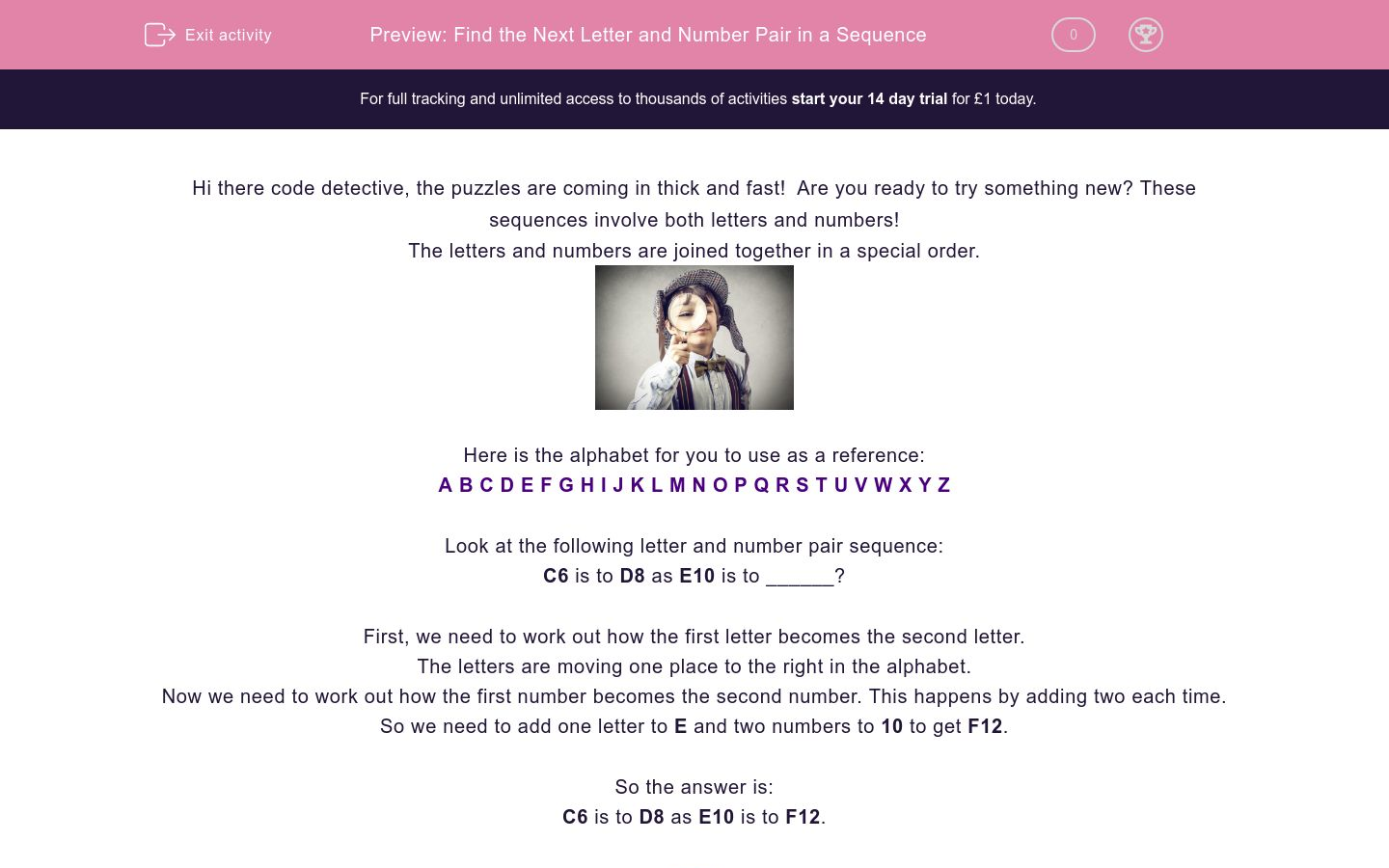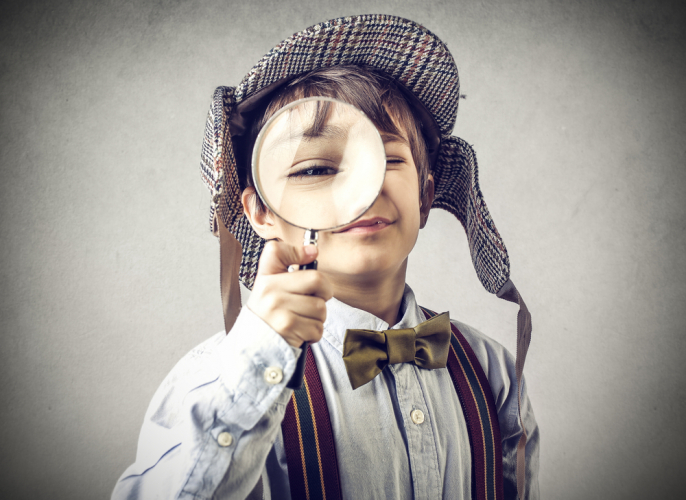# Find the Next Letter and Number Pair in a Sequence

In this worksheet, students will solve sequences involving combined letter and number pairs, with more complex steps, using addition and forward motion. It will develop students’ problem solving and sequencing skills.Key stage:  KS 2

Curriculum topic:   Verbal Reasoning

Curriculum subtopic:   Letter Connections

Difficulty level:### QUESTION 1 of 10

Hi there code detective, the puzzles are coming in thick and fast!  Are you ready to try something new? These sequences involve both letters and numbers!

The letters and numbers are joined together in a special order.Here is the alphabet for you to use as a reference:

A B C D E F G H I J K L M N O P Q R S T U V W X Y Z

Look at the following letter and number pair sequence:

C6 is to D8 as E10 is to ______?

First, we need to work out how the first letter becomes the second letter.

The letters are moving one place to the right in the alphabet.

Now we need to work out how the first number becomes the second number. This happens by adding two each time.

So we need to add one letter to E and two numbers to 10 to get F12.

C6 is to D8 as E10 is to F12.Let’s try another:

G7 is to K9 as S12 is to ______?

First, we need to work out how the first letter becomes the second letter.

The letters are moving four places to the right in the alphabet.

Now we need to work out how the first number becomes the second number. This is adding two each time.

So we need to add four letters to S and two numbers to 12 to get W14.

G7 is to K9 as S12 is to W14.

In this activity, you will need to be sequence superstar to complete the number and letter pairs.

Remember to use the alphabet line to help you work out the code.

Here are two groups of letter and number sequences. Each group follows the same special sequence, but with different letters and numbers.

B42 is to A45 as D50 is to ______ .Use your detective skills to work out the special sequence and find the missing letter and number.

 A  B  C  D  E  F  G  H  I  J  K  L  M  N  O  P  Q  R  S  T  U  V  W  X  Y  Z

Here are two groups of letter and number sequences. Each group follows the same special sequence, but with different letters and numbers.

H70 is to G81 as P45 is to ______ ?A  B  C  D  E  F  G  H  I  J  K  L  M  N  O  P  Q  R  S  T  U  V  W  X  Y  Z

Here are two groups of letter and number sequences. Each group follows the same special sequence, but with different letters and numbers.

T85 is to W74 as Z70 is to ______ ?

 A  B  C  D  E  F  G  H  I  J  K  L  M  N  O  P  Q  R  S  T  U  V  W  X  Y  Z

Here are two groups of letter and number sequences. Each group follows the same special sequence, but with different letters and numbers.

W40 is to T53 as Q73 is to ______ ?A  B  C  D  E  F  G  H  I  J  K  L  M  N  O  P  Q  R  S  T  U  V  W  X  Y  Z

Here are two groups of letter and number sequences. Each group follows the same special sequence, but with different letters and numbers.

F66 is to P78 as T54 is to ______ ?

 A  B  C  D  E  F  G  H  I  J  K  L  M  N  O  P  Q  R  S  T  U  V  W  X  Y  Z

Here are two groups of numbers. Each group follows the same special sequence, but with different numbers.

V50 is to P36 as M70 is to ______ ?A  B  C  D  E  F  G  H  I  J  K  L  M  N  O  P  Q  R  S  T  U  V  W  X  Y  Z

Here are two groups of letter and number sequences. Each group follows the same special sequence, but with different letters and numbers.

M82 is to H97 as F38 is to ______ ?

 A  B  C  D  E  F  G  H  I  J  K  L  M  N  O  P  Q  R  S  T  U  V  W  X  Y  Z

Here are two groups of letter and number sequences. Each group follows the same special sequence, but with different letters and numbers.

S71 is to C57 as R67 is to ______ ?

 A  B  C  D  E  F  G  H  I  J  K  L  M  N  O  P  Q  R  S  T  U  V  W  X  Y  Z

Here are two groups of letter and number sequences. Each group follows the same special sequence, but with different letters and numbers.

N67 is to I80 as G55 is to ______ ?A  B  C  D  E  F  G  H  I  J  K  L  M  N  O  P  Q  R  S  T  U  V  W  X  Y  Z

Here are two groups of letter and number sequences. Each group follows the same special sequence, but with different letters and numbers.

T100 is to O85 as R75 is to ______ ?

 A  B  C  D  E  F  G  H  I  J  K  L  M  N  O  P  Q  R  S  T  U  V  W  X  Y  Z

• Question 1

Here are two groups of letter and number sequences. Each group follows the same special sequence, but with different letters and numbers.

B42 is to A45 as D50 is to ______ .Use your detective skills to work out the special sequence and find the missing letter and number.

 A  B  C  D  E  F  G  H  I  J  K  L  M  N  O  P  Q  R  S  T  U  V  W  X  Y  Z
C53
EDDIE SAYS
Hi there, detective! There are two sequences here. The letter sequence follows the alphabet backwards one jump. The number sequence is add three.
Top tip: The letters can jump forwards or backwards and the number sequence can be addition or subtraction.
• Question 2

Here are two groups of letter and number sequences. Each group follows the same special sequence, but with different letters and numbers.

H70 is to G81 as P45 is to ______ ?A  B  C  D  E  F  G  H  I  J  K  L  M  N  O  P  Q  R  S  T  U  V  W  X  Y  Z
O56
EDDIE SAYS
How are you doing? Have you remembered to look for the relationship between the letters and then the numbers? The letter sequence is to make one one jump backwards in the alphabet. The number sequence is to add eleven. Remember to use the alphabet chart if you need to.
• Question 3

Here are two groups of letter and number sequences. Each group follows the same special sequence, but with different letters and numbers.

T85 is to W74 as Z70 is to ______ ?

 A  B  C  D  E  F  G  H  I  J  K  L  M  N  O  P  Q  R  S  T  U  V  W  X  Y  Z
C59
EDDIE SAYS
Did you work this one out? The letter sequence here is to take three jumps forwards in the alphabet. The number sequence is to subtract eleven.
Top tip: Use a piece of paper to do your working out if you need to!
• Question 4

Here are two groups of letter and number sequences. Each group follows the same special sequence, but with different letters and numbers.

W40 is to T53 as Q73 is to ______ ?A  B  C  D  E  F  G  H  I  J  K  L  M  N  O  P  Q  R  S  T  U  V  W  X  Y  Z

N86
EDDIE SAYS
Did you find the missing letter and number? Don't forget to use the alphabet line. The letter sequence is to make three jumps backwards in the alphabet. The number sequence is to add thirteen.
• Question 5

Here are two groups of letter and number sequences. Each group follows the same special sequence, but with different letters and numbers.

F66 is to P78 as T54 is to ______ ?

 A  B  C  D  E  F  G  H  I  J  K  L  M  N  O  P  Q  R  S  T  U  V  W  X  Y  Z

D66
EDDIE SAYS
Super-cool effort! The letter sequence is to make ten jumps forwards in the alphabet. The number sequence is to add twelve.
• Question 6

Here are two groups of numbers. Each group follows the same special sequence, but with different numbers.

V50 is to P36 as M70 is to ______ ?A  B  C  D  E  F  G  H  I  J  K  L  M  N  O  P  Q  R  S  T  U  V  W  X  Y  Z
G56
EDDIE SAYS
The letter sequence is to make six jumps backwards in the alphabet. The number sequence is to take away fourteen.
With all these sequences your solving, you're becoming a letter and number expert!
• Question 7

Here are two groups of letter and number sequences. Each group follows the same special sequence, but with different letters and numbers.

M82 is to H97 as F38 is to ______ ?

 A  B  C  D  E  F  G  H  I  J  K  L  M  N  O  P  Q  R  S  T  U  V  W  X  Y  Z
A53
EDDIE SAYS
How are you getting on with these?
The letter sequence is to make five jumps backwards in the alphabet. The number sequence is to add fifteen. Great!
• Question 8

Here are two groups of letter and number sequences. Each group follows the same special sequence, but with different letters and numbers.

S71 is to C57 as R67 is to ______ ?

 A  B  C  D  E  F  G  H  I  J  K  L  M  N  O  P  Q  R  S  T  U  V  W  X  Y  Z

B53
EDDIE SAYS
The letter sequence here is to make ten jumps forwards in the alphabet. The number sequence is to subtract fourteen. Super!
• Question 9

Here are two groups of letter and number sequences. Each group follows the same special sequence, but with different letters and numbers.

N67 is to I80 as G55 is to ______ ?A  B  C  D  E  F  G  H  I  J  K  L  M  N  O  P  Q  R  S  T  U  V  W  X  Y  Z
B68
EDDIE SAYS
The letter sequence is to make five jumps backwards in the alphabet. The number sequence is to add thirteen.
One question to go, detective!
• Question 10

Here are two groups of letter and number sequences. Each group follows the same special sequence, but with different letters and numbers.

T100 is to O85 as R75 is to ______ ?

 A  B  C  D  E  F  G  H  I  J  K  L  M  N  O  P  Q  R  S  T  U  V  W  X  Y  Z

M60
EDDIE SAYS
The letter sequence is to make five jumps backwards in the alphabet. The number sequence is to take away fifteen.
Hooray! You've discovered all the letters and numbers that had gone missing. Thanks for all your help!
---- OR ----

Sign up for a £1 trial so you can track and measure your child's progress on this activity.

### What is EdPlace?

We're your National Curriculum aligned online education content provider helping each child succeed in English, maths and science from year 1 to GCSE. With an EdPlace account you’ll be able to track and measure progress, helping each child achieve their best. We build confidence and attainment by personalising each child’s learning at a level that suits them.

Get started